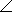# Electrical Engineering - RC Circuits - Discussion

### Discussion :: RC Circuits - General Questions (Q.No.26)

26.

A capacitor with 150of capacitive reactance is across an ac source. The impedance, expressed in polar form, is

 [A]. Z = 150 [B]. Z = 15090°[C]. Z = 150–90°[D]. Z = 150180°Explanation:

No answer description available for this question.

 Satya Prakash said: (Oct 22, 2011) Its very simple 1/jwc Since j is in denominator it will lag by angle=-90degree

 Deepesh Soni said: (Apr 5, 2013) Current lead in capacitor by 90 so impedance will become -90.

 Amit said: (Mar 10, 2014) Why it is -90 it should be +90?

 Arslan Zahoor said: (Jan 19, 2015) Current is leading to voltage 90 degree in capacitor and current is lagging to voltage 90 degree in inductor.

 Haris said: (Jan 11, 2020) Z = R-jxc as R which is not given, it's negligible Z = -j150. -For polar; r = √ (x^2+y^2), r = √ (+(-150)^2), r = 150, ∠= tangent inverse(y/x), =tan.inv(150), = 90 = -90 and sign - because in capacitive impedance lags by 90.